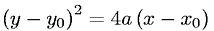Equations > Geometry > Curves and Shapes > Equation of a Parabola

### Equation of a ParabolaLatex Code:

MathML Code:

 ${\left(y-{y}_{0}\right)}^{2}=4a\left(x-{x}_{0}\right)$

MathType 5.0: